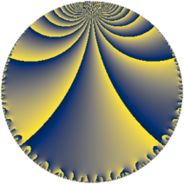# Properties

 Label 76.6.iLevel $76$ Weight $6$ Character orbit 76.i Rep. character $\chi_{76}(5,\cdot)$ Character field $\Q(\zeta_{9})$ Dimension $48$ Newform subspaces $1$ Sturm bound $60$ Trace bound $0$

# Related objects

## Defining parameters

 Level: $$N$$ $$=$$ $$76 = 2^{2} \cdot 19$$ Weight: $$k$$ $$=$$ $$6$$ Character orbit: $$[\chi]$$ $$=$$ 76.i (of order $$9$$ and degree $$6$$) Character conductor: $$\operatorname{cond}(\chi)$$ $$=$$ $$19$$ Character field: $$\Q(\zeta_{9})$$ Newform subspaces: $$1$$ Sturm bound: $$60$$ Trace bound: $$0$$

## Dimensions

The following table gives the dimensions of various subspaces of $$M_{6}(76, [\chi])$$.

Total New Old
Modular forms 318 48 270
Cusp forms 282 48 234
Eisenstein series 36 0 36

## Trace form

 $$48q + 33q^{3} - 177q^{7} + 33q^{9} + O(q^{10})$$ $$48q + 33q^{3} - 177q^{7} + 33q^{9} - 237q^{11} + 2049q^{13} + 2085q^{15} - 609q^{17} - 6012q^{19} + 3591q^{21} + 14100q^{23} + 11802q^{25} - 861q^{27} - 10575q^{29} - 6546q^{31} - 21234q^{33} - 231q^{35} + 20052q^{37} + 72204q^{39} - 3249q^{41} - 34677q^{43} - 34956q^{45} + 4461q^{47} - 41139q^{49} + 12099q^{51} - 24291q^{53} - 61767q^{55} - 64470q^{57} - 20100q^{59} + 95490q^{61} + 86403q^{63} - 57915q^{65} - 64452q^{67} - 99315q^{69} - 115536q^{71} - 16362q^{73} + 236250q^{75} + 26688q^{77} + 29799q^{79} + 180327q^{81} + 52347q^{83} + 204618q^{85} + 69414q^{87} + 47394q^{89} - 249384q^{91} - 462126q^{93} + 412869q^{95} - 229974q^{97} - 692274q^{99} + O(q^{100})$$

## Decomposition of $$S_{6}^{\mathrm{new}}(76, [\chi])$$ into newform subspaces

Label Dim. $$A$$ Field CM Traces $q$-expansion
$$a_2$$ $$a_3$$ $$a_5$$ $$a_7$$
76.6.i.a $$48$$ $$12.189$$ None $$0$$ $$33$$ $$0$$ $$-177$$

## Decomposition of $$S_{6}^{\mathrm{old}}(76, [\chi])$$ into lower level spaces

$$S_{6}^{\mathrm{old}}(76, [\chi]) \cong$$ $$S_{6}^{\mathrm{new}}(19, [\chi])$$$$^{\oplus 3}$$$$\oplus$$$$S_{6}^{\mathrm{new}}(38, [\chi])$$$$^{\oplus 2}$$